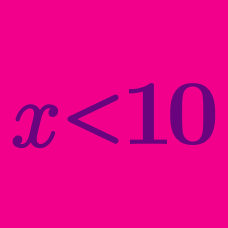Algebra

# Linear Inequalities Word Problems

Consider two successive even numbers. If 10 is subtracted from 4 times the smaller one, then the resulting value is smaller than 2 times the larger one. What is the maximum possible value of the sum of the two successive even numbers?

Adam took an exam with $20$ questions, and answered all of the $20$ questions. He gets $5$ points for a right answer and $2$ points taken off for a wrong answer. If Adam's score in the exam is between $45$ and $80$ exclusive, what is the difference between the maximum possible number of right answers $(M)$ and minimum possible number of right answers $(m)?$

A pair of jeans at Kim's Clothing which was priced $30$% above the merchant's cost is now on sale for $20$% off. At the sale price, the profit margin per unit is at least $$7$. If the merchant's cost of a pair of jeans is at least$$x$, what is $x$?

Let $200n(400-n)$ represent the total revenue (in dollars) of a company from selling $n$ units of the machine they produce, where $0\le n \le 200.$ If it costs $30000$ dollars to produce one unit of the machine and the company wants their profit to be at least $2625000$ dollars, what is the range of the number of units they need to sell?

The results of Richard's first two math tests are $81$ and $84$ points, respectively. What is the minimum score he can achieve on the third test in order to obtain an average of at least $84$ points for all three tests?

×